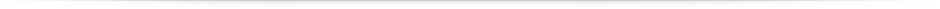# Methodology for the determination of human respiration rate by using Doppler radar and Empirical Modal Decomposition

In this section, we show the results obtained by implementing the methodologies proposed in the previous section where BI and BQ signals are obtained with the radar operating at 4 GHz.

### Experimental results of the methodology to obtain the person’s breathing rate from signals affected by RBM

In the first stage, the capturing of BI and BQ signals were performed, subsequently, the low pass filter was implemented to eliminate high-frequency noise of BI and BQ. In the third stage, the DC offset was removed and the DACM algorithm was subsequently implemented to perform the demodulation process. Finally, the EMD, code available in (ref19), was applied to the phase signal.

Once the EMD method was applied to the signal phase and following the sixth stage of the proposed methodology, the RBM detection process was carried out. For this, the $$A_{max}$$ of the IMF1 is calculated and compared to $$v_{u} = 0.7$$ to decide whether movement exists. Figure 3a shows the phase signal for two cases where there is a head (H) movement, two cases where there is an arm (A) movement, and one case in which a movement combination of arms, legs, and torso (M) was carried out. Figure 3b shows the IMF1 of the EMD for each of the movements performed by the PUT. Figure 3b shows that IMF1 contains enough information to detect any RBM made by the PUT. It is also important to mention that $$v_{u} = 0.7$$ value was obtained by 30 measurements with the PUT at rest; 0.7 being the maximum value obtained from the measurements.

### Experimental results of both measure breathing rates methodology and CC-drift/RBM elimination methodology

This methodology uses the IMFs obtained with the EMD. Figure 4a and b show the raw experimental results of the phase signal and spectrogram obtained from the person under test (PUT) at rest. In Fig. 4a the presence of CC can be observed, which is most noticeable in the spectrogram plotted in Fig. 4b. As it can be seen, there is a concentration of power around the frequency 0 Hz at any moment of time. Figure 4c and d show the experimental results of the phase signal and spectrogram corrected with the proposed methodology. Figure 4c shows that the corrected signal does not have a CC, confirmed by its spectrogram shown in Fig. 4d. In this Figure, the components around the frequency 0 Hz have low power while there is an important power spectral density (PSD) magnitude around 0.35 Hz, corresponding to 21 breaths/min.

Figure 5 shows the results obtained after two experiments in which the PUT moves the head perpendicular to its chest. Figure 5a shows that before correction, there is a significant PSD magnitude around the frequency 0 Hz, and at 15 s, the head movement carried out adds CC drift to the phase signal; this can be observed in the spectrogram as an increase in the low-frequency PSD. It is important to mention that in the same spectrogram can be seen the generation of spurious at high frequencies, which are due to the random nature of head movement. Following the application of the proposed methodology, the spectrogram in Fig. 5b shows the mitigation of spurious signals (CC, CC drift and high frequency signals) and that the breathing rate is around 0.35 Hz, which corresponds to 20.82 breaths/min.

On the other hand, Fig. 5c and d show the spectrogram before and after correction, which are the result of the second head movement experiment. The PSD around the frequency of 0 Hz can be observed in the spectrogram in Fig. 5c. In addition, approximately after 25 s, there is a head movement, which adds CC drift and spurious of considerable power. Applying the proposed methodology, we obtain the spectrogram in Fig. 5d, which clearly shows the mitigation of spurious signals and a breathing rate of around 0.30 Hz which corresponds to 18.19 breaths/min.

Figure 6 shows the results of two experiments when PUT performs movement in the left arm until it is perpendicular to its chest. Figure 6a shows the spectrogram of the raw phase signal where it is always observed that there is a concentration of power around the frequency 0 Hz; In addition, approximately, when time is equal to 15 s, there is arm movement, which generates CC drift and spurious at high frequencies. On the other hand, Fig. 6b shows that the proposed methodology significantly mitigates spurious signals and that the breathing rate is around 0.39 Hz which corresponds to 23.44 breaths/min.